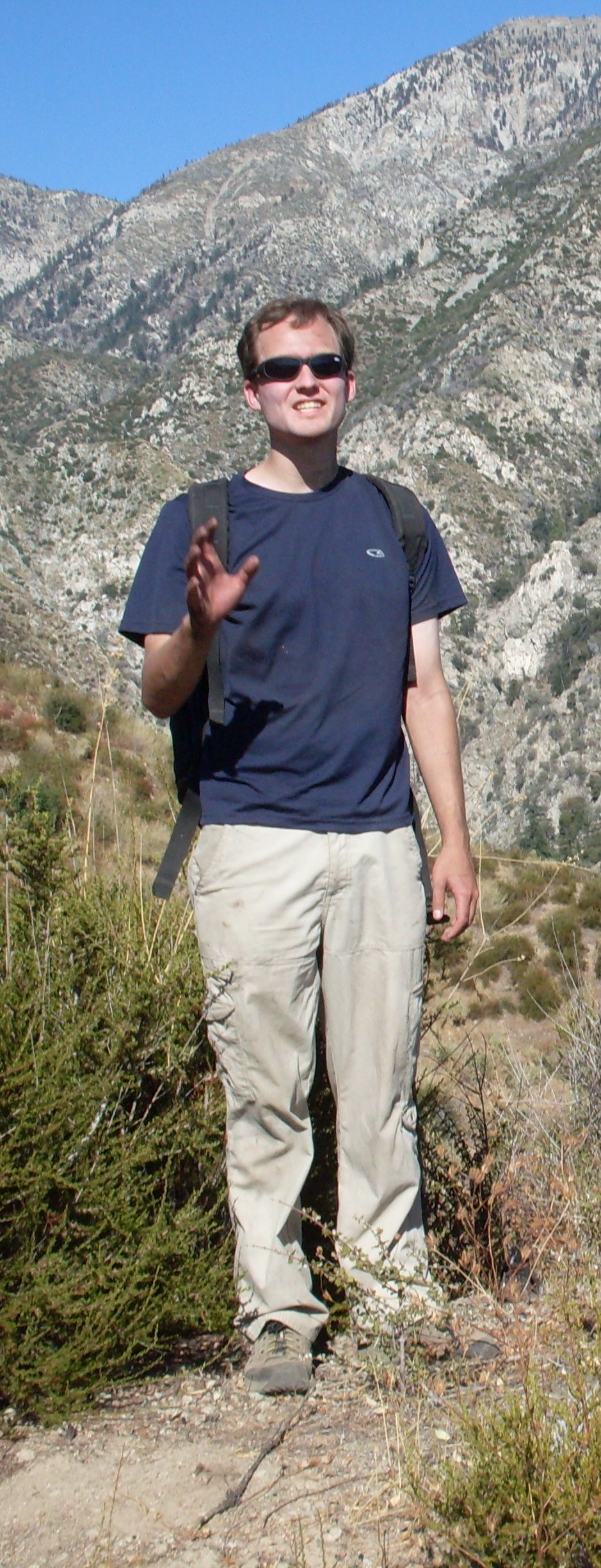I have enjoyed having the opportunity to teach a variety of courses at the University of Oklahoma. By term, here is what I taught:

In Spring 2014, I taught Sections 001 and 900 of MATH 2443 (Calculus and Analytic Geometry IV).

In Fall 2013, I taught Section 002 of MATH 3333 (Linear Algebra I).

In Spring 2013, I taught Section 002 of MATH 3113 (Introduction to Ordinary Differential Equations).

In Fall 2012, I taught Section 009 of MATH 2433 (Calculus and Analytic Geometry III) and Section 002 of MATH 3113 (Introduction to Ordinary Differential Equations).

In Spring 2012, I taught Sections 001 and 002 of MATH 3113 (Introduction to Ordinary Differential Equations).

In Fall 2011, I taught Section 900 of MATH 2433 (Calculus and Analytic Geometry III).

Department of Mathematics
The University of Oklahoma
Norman, OK 73019-3103
(626) 200-9460
jlouwsma AT ou DOT edu
Physical Sciences Center 1007